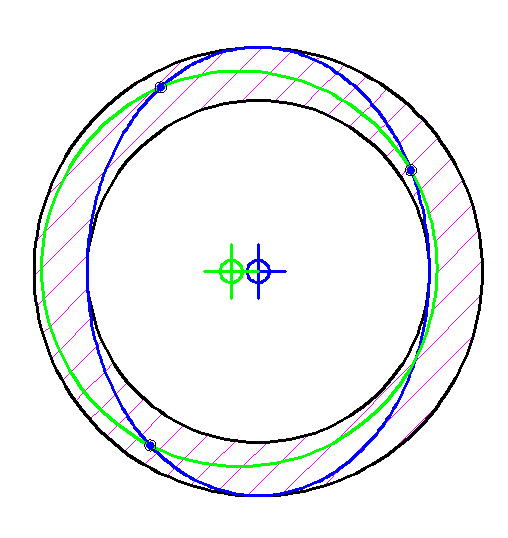# Measurement of concentricity using CMM

• k.udhay

#### k.udhay

Hello,

If I want to measure the concentricity of a cylinder with another, is it possible using a CMM? If yes, can you pl. explain me the procedure?

I have read that concnetricity has to be measured based on the mid point of a diameter-line of a cylinder. This means, I have to measure the co-ordinates of two exact opposite points in the circumference of the cylinder. And then, I have to repeat this for atleast 3 (?) different diameter-lines. How can I do this in CMM without any arrangement to index the job exactly by 180°? I hope I have explained the portion of my confusion here. Kindly help me out!

Thanks.

If you measure three points arbitrarily spaced around each end of each cylinder, you will be able to fit a circle to each of those four sets of three points, in 3D.

Compute the coordinates of those four circle's centres.
You now have four points that should lie close to a common straight line.
Compute the concentricity from the deviation of those points from the straight line.

•1 person
Thanks, Baluncore.
But, won't this method be very approximate to measure concentricity?
Let us assume this way:
I have got a circular hole given a position tolerance with an MMC. Hence, even if the hole become little elliptical but still accepting the go and no-go gauges, the position tolerance is met. I think the CMM way of measuring this also will give me the same conclusion. But, if this was concentricity, still I can accept this, as concentricity doesn't mind if this hole was elliptical, unless it's axis lies on the tolerated boundary. But the CMM drawn circle will say that the hole is eccentric to the datum which is not true...In the above image, the shaded portion shows the VC of the hole. The blue ellipse is the actual hole machined. There are three arbitrary points on the hole touched by CMM probe. Green is the CMM drawn circle, which is acceptable as per position tolerance. But it's centre is away from the true centre of the ellipse and hence may be rejected by concentricity tolerance...
What is your opinion on this?

Last edited:
Your OP specified the concentricity of one cylinder with another. You have now replaced the perfect cylinders with elliptical section holes.

That could be because you are not measuring on a plane perpendicular to their axis but on a slight diagonal.
If you align your cylinders close to a machine axis, the elliptical error will be a cosine function.
A one degree alignment error will change your radius estimate by a maximum of 1 – Cos( 1° ) = 0.00015
That should attenuate any elliptical effect by 6500 times or more, so maybe no problem.

A circle has a constant radius with direction. It takes three measurements to find the centre. Sampling theory says you must sample a signal at twice the rate of the highest frequency present. An ellipse has a 2'nd harmonic radius. (It has two minima and two maxima). The frequency of two requires four sample points to fit a Fourier ellipse approximation. But can the ellipse be fitted and the centre found with 4 points or does it take more? What if the points are arbitrarily spaced rather than equally spaced?

If the holes are drilled or milled with a drill having n cutting edges then they will have a radial frequency of n+1. A two flute drill produces slightly triangular holes. To bore an accurate cylindrical hole is a special case, you must use a single cutting edge that is the only point of contact.

If your holes are so elliptical that they intrude on the positional tolerance then you need to drill rounder holes.
An elliptical hole is usually only produced by different rigidity or movement in an x or y machine way.

Last edited:
•1 person